# 架构师训练营 第五周 作业﻿

# 哈希算法

﻿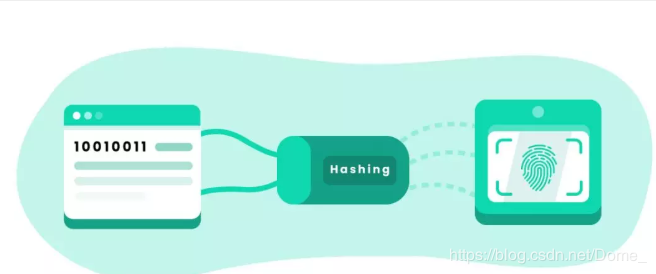﻿

﻿

﻿

﻿

﻿

﻿

﻿

# 一致性哈希算法

﻿

﻿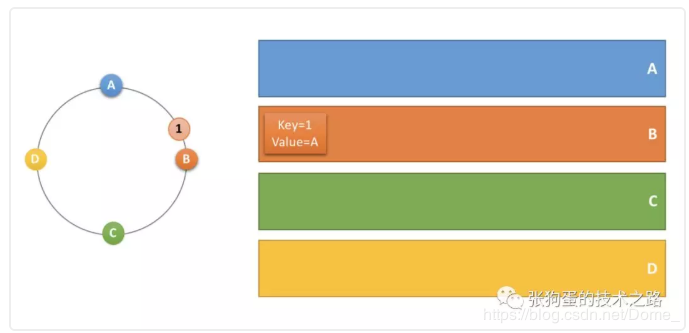﻿

﻿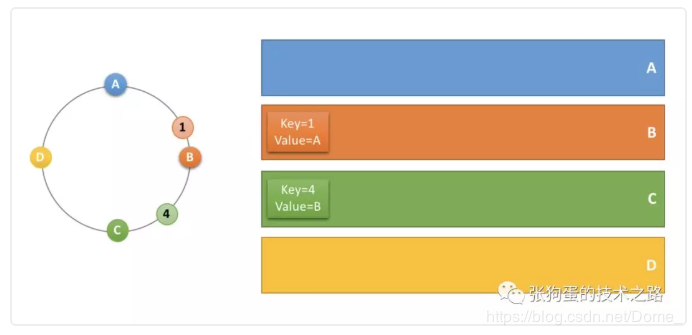﻿

﻿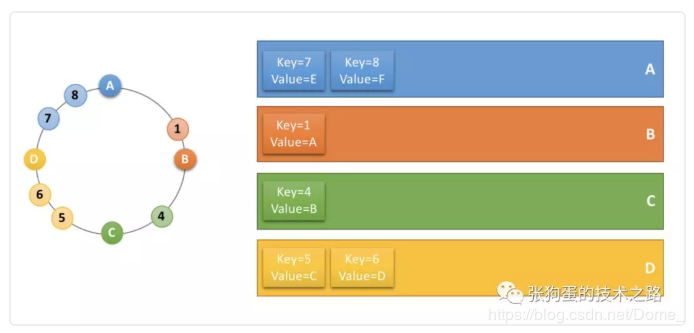﻿

﻿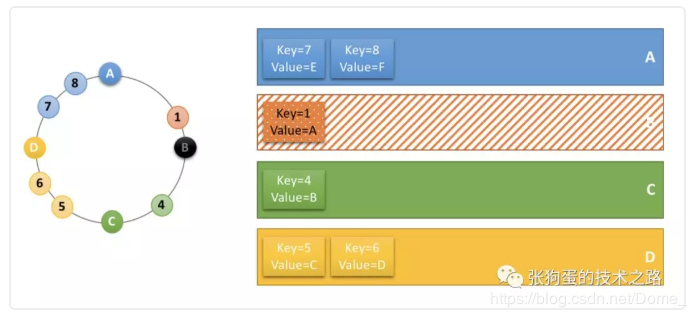﻿

﻿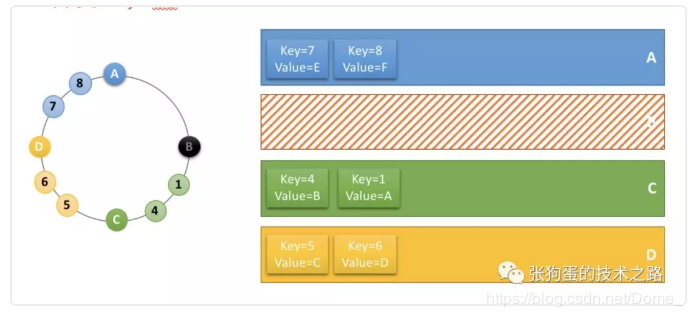﻿

﻿

﻿

StatisticsUtil

public class StatisticsUtil {    /**     * //方差s^2=[(x1-x)^2 +...(xn-x)^2]/n     * @return     * @exception     * @author lvq     * @date 2020/7/8 18:58     */    public static double variance(Long[] x) {        int m = x.length;        double sum = 0;        for (int i = 0; i < m; i++) {//求和            sum += x[i];        }        double dAve = sum / m;//求平均值        double dVar = 0;        for (int i = 0; i < m; i++) {//求方差            dVar += (x[i] - dAve) * (x[i] - dAve);        }        return dVar / m;    }    /**     * //标准差σ=sqrt(s^2)     * @return     * @exception     * @author lvq     * @date 2020/7/8 18:58     */    public static double standardDeviation(Long[] x) {        int m = x.length;        double sum = 0;        for (int i = 0; i < m; i++) {//求和            sum += x[i];        }        double dAve = sum / m;//求平均值        double dVar = 0;        for (int i = 0; i < m; i++) {//求方差            dVar += (x[i] - dAve) * (x[i] - dAve);        }        return Math.sqrt(dVar / m);    }}

﻿

KeyUtil

public class KeyUtil{    private KeyUtil() {        // Empty    }    /**     * @return     * @exception     * @author lvq     * @param  k     * @date 2020/7/7 20:40     */    public static byte[] getKeyBytes(String k) {        try {            return k.getBytes("UTF-8");        } catch (UnsupportedEncodingException e) {            throw new RuntimeException(e);        }    }    /**     * @return     * @exception     * @author lvq     * @param  keys     * @date 2020/7/7 20:41     */    public static Collection<byte[]> getKeyBytes(Collection<String> keys) {        Collection<byte[]> rv = new ArrayList<byte[]>(keys.size());        for (String s : keys) {            rv.add(getKeyBytes(s));        }        return rv;    }}

﻿

HashAlgorithm.java

public interface  HashAlgorithm {    long hash(final String k);}

﻿

DefaultHashAlgorithm

public enum DefaultHashAlgorithm implements HashAlgorithm {    /**     * Native hash (String.hashCode()).     */    NATIVE_HASH,    /**     * CRC_HASH as used by the perl API. This will be more consistent both     * across multiple API users as well as java versions, but is mostly likely     * significantly slower.     */    CRC_HASH,    /**     * FNV hashes are designed to be fast while maintaining a low collision rate.     * The FNV speed allows one to quickly hash lots of data while maintaining a     * reasonable collision rate.     *     * @see <a href="http://www.isthe.com/chongo/tech/comp/fnv/">fnv     *      comparisons</a>     * @see <a href="http://en.wikipedia.org/wiki/Fowler_Noll_Vo_hash">fnv at     *      wikipedia</a>     */    FNV1_64_HASH,    /**     * Variation of FNV.     */    FNV1A_64_HASH,    /**     * 32-bit FNV1.     */    FNV1_32_HASH,    /**     * 32-bit FNV1a.     */    FNV1A_32_HASH,    /**     * MD5-based hash algorithm used by ketama.     */    KETAMA_HASH,    MURMUR_HASH;    private static final long FNV_64_INIT = 0xcbf29ce484222325L;    private static final long FNV_64_PRIME = 0x100000001b3L;    private static final long FNV_32_INIT = 2166136261L;    private static final long FNV_32_PRIME = 16777619;    private static MessageDigest md5Digest = null;    static {        try {            md5Digest = MessageDigest.getInstance("MD5");        } catch (NoSuchAlgorithmException e) {            throw new RuntimeException("MD5 not supported", e);        }    }    /**     * @return     * @exception     * @author lvq     * @param  k     * @date 2020/7/7 20:42     */    @Override    public long hash(final String k) {        long rv = 0;        int len = k.length();        switch (this) {            case NATIVE_HASH:                rv = k.hashCode();                break;            case CRC_HASH:                // return (crc32(shift) >> 16) & 0x7fff;                CRC32 crc32 = new CRC32();                crc32.update(KeyUtil.getKeyBytes(k));                rv = (crc32.getValue() >> 16) & 0x7fff;                break;            case FNV1_64_HASH:                rv = FNV_64_INIT;                for (int i = 0; i < len; i++) {                    rv *= FNV_64_PRIME;                    rv ^= k.charAt(i);                }                break;            case FNV1A_64_HASH:                rv = FNV_64_INIT;                for (int i = 0; i < len; i++) {                    rv ^= k.charAt(i);                    rv *= FNV_64_PRIME;                }                break;            case FNV1_32_HASH:                rv = FNV_32_INIT;                for (int i = 0; i < len; i++) {                    rv *= FNV_32_PRIME;                    rv ^= k.charAt(i);                }                break;            case FNV1A_32_HASH:                rv = FNV_32_INIT;                for (int i = 0; i < len; i++) {                    rv ^= k.charAt(i);                    rv *= FNV_32_PRIME;                }                break;            case KETAMA_HASH:                byte[] bKey = computeMd5(k);                rv = ((long) (bKey & 0xFF) << 24)                        | ((long) (bKey & 0xFF) << 16)                        | ((long) (bKey & 0xFF) << 8)                        | (bKey & 0xFF);                break;            case MURMUR_HASH:                rv = murmurHash(k);                break;            default:                assert false;        }        return rv & 0xffffffffL; /* Truncate to 32-bits */    }    private long murmurHash(String key) {        ByteBuffer buf = ByteBuffer.wrap(KeyUtil.getKeyBytes(key));        int seed = 0x1234ABCD;        ByteOrder byteOrder = buf.order();        buf.order(ByteOrder.LITTLE_ENDIAN);        long m = 0xc6a4a7935bd1e995L;        int r = 47;        long h = seed ^ (buf.remaining() * m);        long k;        while (buf.remaining() >= 8) {            k = buf.getLong();            k *= m;            k ^= k >>> r;            k *= m;            h ^= k;            h *= m;        }        if (buf.remaining() > 0) {            ByteBuffer finish = ByteBuffer.allocate(8).order(                    ByteOrder.LITTLE_ENDIAN);            finish.put(buf).rewind();            h ^= finish.getLong();            h *= m;        }        h ^= h >>> r;        h *= m;        h ^= h >>> r;        buf.order(byteOrder);        return h;    }    public static byte[] computeMd5(String k) {        MessageDigest md5;        try {            md5 = (MessageDigest) md5Digest.clone();        } catch (CloneNotSupportedException e) {            throw new RuntimeException("clone of MD5 not supported", e);        }        md5.update(KeyUtil.getKeyBytes(k));        return md5.digest();    }}

﻿

ServerNode

public class ServerNode {    private final SocketAddress socketAddress;    /**     * @return     * @exception     * @author lvq     * @param  socketAddress     * @date 2020/7/7 20:20     */    public ServerNode(SocketAddress socketAddress)    {        this.socketAddress=socketAddress;    }    /**     * @return     * @exception     * @author lvq     * @date 2020/7/7 20:20     */    public SocketAddress getSocketAddress()    {        return socketAddress;    }}

﻿

NodeLocator

public interface NodeLocator {     /**      * @return      * @exception      * @author lvq      * @param  s      * @date 2020/7/7 20:17      */    ServerNode getPrimary(String s);}

ConsistentHashNodeLocator

public class ConsistentHashNodeLocator implements NodeLocator {    private final static int VIRTUAL_NODE_SIZE = 12;    private final  static String VIRTUAL_NODE_SUFFIX = "-";    private volatile TreeMap<Long,ServerNode> hashRing;    private final HashAlgorithm hashAlg;    /**     * @return     * @exception     * @author lvq     * @param  nodes     * @param  hashAlg     * @date 2020/7/7 20:29     */    public ConsistentHashNodeLocator(List<ServerNode> nodes,HashAlgorithm hashAlg)    {        this.hashRing=buildConsistentHashRing(hashAlg, nodes);        this.hashAlg = hashAlg;    }    /**     * @return     * @exception     * @author lvq     * @param  hashAlg     * @param  nodes     * @date 2020/7/7 20:28     */    private TreeMap<Long, ServerNode> buildConsistentHashRing(HashAlgorithm hashAlg, List<ServerNode> nodes)    {        TreeMap<Long, ServerNode> virtualNodeRing = new TreeMap<Long, ServerNode>();        for (ServerNode node : nodes) {            for (int i = 0; i < VIRTUAL_NODE_SIZE; i++) {                // 新增虚拟节点的方式如果有影响，也可以抽象出一个由物理节点扩展虚拟节点的类                virtualNodeRing.put(hashAlg.hash(node.getSocketAddress().toString() + VIRTUAL_NODE_SUFFIX + i), node);            }        }        return virtualNodeRing;    }    /**     * @return     * @exception     * @author lvq     * @param  * @param null     * @date 2020/7/7 20:31     */    @Override    public ServerNode getPrimary(String s)    {        long hash=hashAlg.hash(s);        return getNodeForkey(hashRing,hash);    }    /**     * @return     * @exception     * @author lvq     * @date 2020/7/7 20:31     */    public ServerNode getNodeForkey(TreeMap<Long, ServerNode> hashRing, long hash)    {        // 向右查找firsrt key        Map.Entry<Long,ServerNode> locatenode=hashRing.ceilingEntry(hash);        //        if (locatenode == null) {            locatenode = hashRing.firstEntry();        }        return locatenode.getValue();    }}

main

public class Main {    public static void main(String[] args)    {        testDistribution();     }    public static void testDistribution()    {        List<ServerNode> servers = new ArrayList<ServerNode>();        for (String ip : ips) {            servers.add(new ServerNode(new InetSocketAddress(ip, 8080)));        }        NodeLocator nodeLocator = new ConsistentHashNodeLocator(servers, DefaultHashAlgorithm.KETAMA_HASH);        // 构造 1000000 随机请求        List<String> keys = new ArrayList<String>();        for (int i = 0; i < 1000000; i++) {            keys.add(UUID.randomUUID().toString());        }        // 统计分布        AtomicLongMap<ServerNode> atomicLongMap = AtomicLongMap.create();        for (ServerNode server : servers) {            atomicLongMap.put(server, 0);        }        for (String key : keys) {            ServerNode node = nodeLocator.getPrimary(key);            atomicLongMap.getAndIncrement(node);        }        System.out.println(StatisticsUtil.variance(atomicLongMap.asMap().values().toArray(new Long[]{})));        System.out.println(StatisticsUtil.standardDeviation(atomicLongMap.asMap().values().toArray(new Long[]{})));    }     static String[] ips = {            "10.238.172.215",            "10.238.176.96",            "10.238.65.34",            "10.238.64.205",            "10.238.65.67",            "10.238.247.206",            "10.238.173.47",            "10.238.65.117",            "10.238.69.32",            "10.238.173.46"    };}

﻿

12个虚拟节点

﻿

100个虚拟节点

﻿

1000个虚拟节点

﻿

﻿## 评论